# Algorithms on Graphs: Let’s talk Depth-First Search (DFS) and Breadth-First Search (BFS)

One thing that we’ll be doing a lot with the algorithms in this series is graph traversal. What does this mean exactly?

Basically, the idea is that we’ll be moving around the graph from one vertex to another and discovering the properties of their interconnected relationships.

Two of the most commonly used algorithms that we’ll use a lot is: Depth-First Search (DFS) and Breadth-First Search (BFS).

While both these algorithms allow us to traverse graphs, they differ in varying ways. Let’s start with DFS.

# Depth-First Search (BFS)

DFS utilizes the “go deep, head first” philosophy in its implementation. The idea is that starting from an initial vertex (or place), we go down one path until we reach the end and if we don’t reach our destination, then we come back and go down a different path.

Let’s look at an example. Suppose we have a directed graph that looks like this:

Now the idea is that we start at vertex s and we’re asked to find vertex t. Using DFS, we’re going to explore one path, go all the way to the end and if we don’t find t, then we go down another path. Here’s the process: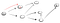Going down the path of the first neighboring vertex

So here, we go down the path (p1) of the first neighboring vertex and we see that it is not the end because it has a path towards another vertex. So we go down that path:

This is clearly not the vertex t so we have to go back because we reached a dead end. Since the previous node doesn’t have anymore neighbors, we go back to s. From s, we go to its second neighboring node:

Going down the path (p2), we’re confronted with three neighbors. Since the first one has already been visited, we have to go down the second one:

Now, once again we’ve reached a dead end that is not the vertex t so we have to go back. Since there’s another neighbor that hasn’t been visited, we go down that neighboring path and at last we found the the vertex t:

This is how DFS works. Go down a path and keep going until you’ve reached the destination or a dead end. If it’s the destination, you’re done. If it’s not, then go back and continue down a different path until you’ve exhausted all options within that path.

We can see that we follow the same procedure at each vertex that we visit:

Do a DFS for each neighbor of the vertex

Since this entails doing the same procedure at each step, something tells us that we’ll need to use recursion to implement this algorithm.

Here’s the code in JavaScript:

Note: This specific DFS algorithm allows us to determine if it’s possible to reach from one place to another. DFS can be used in a variety of ways and there may be subtle changes to the algorithm above. However the general concept remains the same.

## Analysis of DFS

Now let’s analyze this algorithm. Since we’re traversing through each neighbor of the node and we’re ignoring the visited ones, we have a runtime of O(V + E).

A quick explanation of exactly what V+E means:

V represents the total number of vertices. E represents the total number of edges. Every vertex has a number of edges.

While it may seem that one may be led to believe that it is V•E instead of V + E, let’s think about what V•E means exactly.

For something to be V•E, it would mean that for each vertex, we have to look at all the edges in the graph regardless of whether or not those edges are connected to that specific vertex.

While, on the other hand, V + E means that for each vertex, we only look at the number of edges that pertain to that vertex. Recall from the previous post, that the space we take up for the adjacency list is O(V + E). Each vertex has a number of edges and in the worst case, if we were to run DFS on each vertex, we would have done O(V) work along with exploring all the edges of the vertices, which is O(E). Once we’ve looked at all V number of vertices, we would have also looked at a total of E edges. Therefore, it is V + E.

Now, since DFS uses recursion on each vertex, that means that a stack is used (which is why it’s called a stack overflow error whenever you run into an infinite recursive call). Therefore, the space complexity is O(V).

Now let’s see how breadth-first search differs.

# Breadth-First Search

Breadth-First Search (BFS) follows the “go wide, bird’s eye-view” philosophy. What that basically means is that instead of going all the way down one path until the end, BFS moves towards its destination one neighbor at a time. Let’s look at what that means: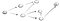same graph as before

So instead of just going all the way down its first neighbor, BFS would visit all the neighbors of s first and then visit those neighbors’ neighbors and so forth until it reaches t. Here’s how it would look: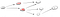it looks at its neighbors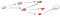then it looks at its neighbors’ neighbors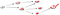then finally finds t

See how different DFS and BFS behave? While I like to think that DFS likes to go head on, BFS likes to look take it slow and observe everything one step at a time.

Now one question that stands out to us should be: “How do we know which neighbors to visit first from s’s neighbors?”

Well, we could utilize a queue’s first-in-first-out (FIFO) property where we pop the first vertex of the queue, add its unvisited neighbors to the queue, and then continue this process until the queue is either empty or the vertex we add to it is the vertex we’ve been looking for.

Now let’s look at the code in JavaScript:

## Analysis of BFS

It may seem like BFS is slower. But if you look carefully at the visualizations of both BFS and DFS, you’ll find that they actually have the same runtime.

The queue ensures that at most every vertex will be processed until it reaches the destination. So that means at the worst case, BFS will also look at all the vertices and all the edges.

While BFS may seem slower, it’s actually deemed faster because if we were to implement them on larger graphs, you’ll find that DFS wastes a lot of time going down long paths that are ultimately wrong. In fact, BFS is often used in algorithms to determine the shortest path from one vertex to another, but we’ll touch on those later.

So since the runtimes are the same, BFS has a runtime of O(V + E) and due to the use of a queue that can hold at most V vertices, it has a space complexity of O(V).

# Analogies to Leave Off With

I want to leave you off with other ways that I personally imagine how DFS and BFS work in hopes that it will help you remember as well.

Whenever I think of DFS, I like to think of something that finds the right path by bumping into a lot of dead ends. Usually, this would be like a mice going through a maze to look for food. It would try a path, find out that the path is a dead end, then pivot to another path and repeat this process until it reaches its target:

And this is what a simplified version of the process would look like:

Now for BFS, I’ve always imagined it as a ripple. A disturbance at the source causes water to push in all directions and creates a chain effect of water molecules pushing neighboring molecules to create a ripple effect:

Much like how BFS starts at the source and visits the source’s neighbors first and then goes more outwards by visiting their neighbors and so on: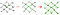Like a ripple

# Summary

• Depth-First Search (DFS) and Breadth-First Search (BFS) are both used to traverse graphs.
• DFS charges down one path until it has exhausted that path to find its target, while BFS ripples through neighboring vertices to find its target.
• DFS uses a stack while BFS uses a queue.
• Both DFS and BFS have a runtime of O(V + E) and a space complexity of O(V).
• Both algorithms have different philosophies but share equal importance in how we traverse graphs.

## More from Try Khov

Software Engineer